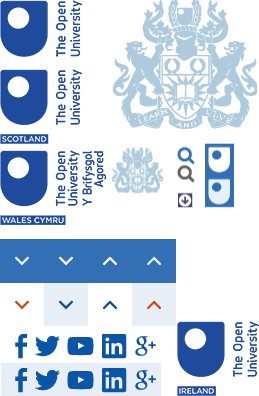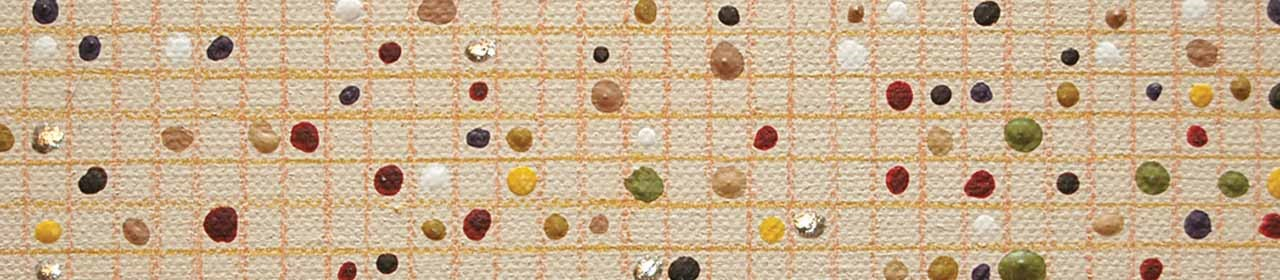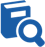﻿ Developing Knowledge | Faculty of Science, Technology, Engineering & Mathematics## You are here

1. Home
2. Study
3. Professional STEM Education Centre
4. Mathematics Education
5. Developing Knowledge

# Developing KnowledgeThe Open University offers several ways to develop your knowledge of mathematics and mathematics education through distance learning.

OpenLearn – for free, short courses that introduce key issues in teaching. These stand-alone resources can be used to guide department discussions and training, or studied on your own.

Mathematics Education Modules - these are substantial, accredited modules at undergraduate level that you can study through distance-learning, supported by a tutor.

On this page, we have included two groups of modules relevant to school mathematics: modules that teach mathematical content (Mathematics and its applications) and modules about teaching and pedagogy in mathematics (Mathematics Education) You can find how to register for these modules here.

### Mathematics and its applications

Anyone can sign up for an OU module to improve their mathematics knowledge, but it is sensible to plan where to start. Are you ready quizzes will help you decide whether you are ready for Discovering mathematics (MU123), or for Essential mathematics (MST124).  If you are thinking about teaching or supporting mathematics, you need to have a confident understanding of those parts of degree-level mathematics that underpin the school curriculum.   The modules that are most relevant to the mathematics taught in school are:Teaching to age 14:

Discovering Mathematics (MU123) looks at a variety of mathematical topics such as numbers, statistics, graphs, algebra, trigonometry and associated techniques. It also introduces mathematical modelling and some problem-solving strategies. As well as ‘doing the maths’ you will learn how to interpret your results in context and to explain your approach and conclusions. This module is ideal for potential teachers who need to start with basic mathematical concepts and build from there. The module contains many worked examples in the form of short videos which can really help you to develop good teaching skills.

Teaching to age 16:

Introducing Statistics (M140) is about using data, usually in the form of numbers, to describe aspects of society and the environment. This module gives many examples of the way statistical thinking can be used to help in important aspects of our lives. In addition, M140 shows the connection to other subjects that can involve statistical ideas, such as economics, business, engineering, and natural and social sciences. Like MU123, this modules starts with basic concepts and uses short videos to compliment the teaching material to develop the idea of statistical thinking. When combined with MU123 (above) you will have covered all the relevant material for GCSE, Core Maths or equivalent and more, but in greater detail in a very structured approach.Teaching to age 18:

Once you move to A-level or equivalent, there are two specific requirements that you will need to fulfil.

• You need a firm understanding of the mathematics that you will be teaching.

• You need to be able to engage the students in mathematical discussion which requires you to have a confident understanding of mathematics at a more advanced level.

Essential Mathematics I (MST124) provides a foundation in the essential mathematical ideas and techniques that underpin the study of mathematics and mathematical subjects such as physics, engineering and economics. Essential Mathematics II (MST125) builds on the mathematical ideas developed in MST124 and students study a wide range of topics from different areas of mathematics and learn how many of them can be applied to practical problems. Essential Mathematics I and Essential Mathematics II cover all the mathematics required to teach pure mathematics and further pure mathematics at A-level and beyond. Both modules employ a formal and detailed approach to learning to ensure that students are well prepared for more advanced mathematics study.

Pure Mathematics (M208) is a level 2 module that builds on the foundations laid in MST124 and MST125 and includes the theoretical foundations of the pure mathematics A-level syllabuses.  Once you have mastered the concepts of mathematical rigour and abstraction, you will have the tools and mathematical confidence to engage your students in mathematical dialogue beyond A-level. Pure mathematics is one of the oldest creative human activities and this module introduces its main topics. Group Theory explores sets of mathematical objects that can be combined – such as numbers, which can be added or multiplied, or Linear Algebra explores 2- and 3-dimensional space and systems of linear equations. Analysis, the foundation of calculus, covers operations such as integration, arising from infinite limiting processes.

Potential teachers who wish to teach other areas of mathematics at A-level for example Statistics, Discrete mathematics or mechanics should consider the following modules:

Analysing Data (M248) explores the fundamental statistical techniques and ideas used for analysing and interpreting data, covering models for data, estimation, confidence intervals, hypothesis testing and regression. The emphasis is on the practical side, although some of the underlying theory is also included. This module is ideal if you would like to develop the skills to make sense of data. It also provides the necessary foundations required for studying further modules in statistics. It builds on M140 (above) and will provide you with a sufficient knowledge of statistics to teach the subject at A-level.Mathematical methods (MST224) is designed to teach you about a variety of mathematical methods which are used in modelling through their application to solving real world problems. These methods include differential equations, linear algebra and vector calculus. You will become familiar with new mathematical skills mainly by using pencil and paper and by thinking. When combined with MST124 and MST125, this module not only covers the A-level Applied Mathematics (mechanics) syllabus but also takes you to the next level so that you can engage in meaningful dialog about the subject with your students.

Graphs, Networks and Design (MT365) is about using ideas from discrete mathematics to model problems, and representing these ideas through diagrams. The word ‘graphs’ refers to diagrams consisting of points joined by lines, that represent any kind of connected structures. The main topics of mathematical interest are graphs and digraphs; network flows; block designs; geometry; codes; and mathematical modelling. Application areas covered include communications; structures and mechanisms; electrical networks; transport systems; social networks; and computer science. This module is ideal for teachers who wish to teach discrete mathematics in Further Maths A-level. It provides you with the necessary subject knowledge to engage your students in interesting discussion about everyday practical real world problems.

### Mathematics Education undergraduate level modules

The OU offers four distance-learning specialist mathematics education modules at level 3. They can be studied as part of a Mathematics and its Learning degree, or as part of an Open degree. They are also ideal standalone modules for those who already hold a non-mathematical degree and who are considering teaching mathematics.

For each of these modules you need to be able to study for 12 hours a week over 6 months, and have access to a small group of learners. These mathematics education modules will give you a deep understanding of how people develop mathematical thinking. Each is supported by undergraduate tutors and assessed through coursework.

### Mathematical Thinking in schools (ME620)

ME620 focuses on mathematics from 11-14. The module offers a series of mathematical tasks and guided reflection activities. You will learn to use key analytic frameworks to reflect on your own work and your learners’ activities, and to identify mathematical thinking processes. You will learn to observe learners, compare their different approaches and responses to the tasks.

### Developing algebraic thinking (ME625)

ME625 provides a detailed analysis of school algebra. Based around mathematical tasks and guided reflection, with tutorial support, it will enable you to think of yourself as a learner of algebra in a new way and thus to teach algebra more successfully. It is aimed at teachers or those interested in working with pupils aged 7-16 and discusses principles that teachers have found useful in preparing lessons.

### Developing geometric thinking (ME627)

ME627 develops understanding of the school geometry curriculum and beyond. Based on the OU principle of mathematical tasks and guided reflection, supported by interactive tutorials, it encourages you to think more deeply about the nature and essence of geometry and its teaching and learning. This module re-establishes geometric thinking in its historical place as a significant means of mathematical perception and reasoning.

### Developing statistical thinking (MK626)

MK626 heightens awareness of statistical ideas and supports students to think statistically about real-life problems using accessible data. It uses the OU principle of mathematical tasks, guided reflection and interactive tutorials to promote discussion of statistical pedagogy with developing knowledge of statistics. The framework offered this module supports the use of large data sets in A-level mathematics.

### Registering for stand-alone modules

If you want to study a single module, rather than a whole degree, please visit the relevant module page and check its cost and its timings. Each page gives details of advisers who can phone and talk you through an application. Click on the Module Cost/Registration link or tab to find the button and give your details.

## Meet our AcademicsIn addition to teaching on Open University modules our academics are engaged in ground breaking research that benefits individuals and society.

## Request your prospectusExplore our qualifications and courses by requesting one of our prospectuses today.

## Are you already an OU student?

Go to StudentHome